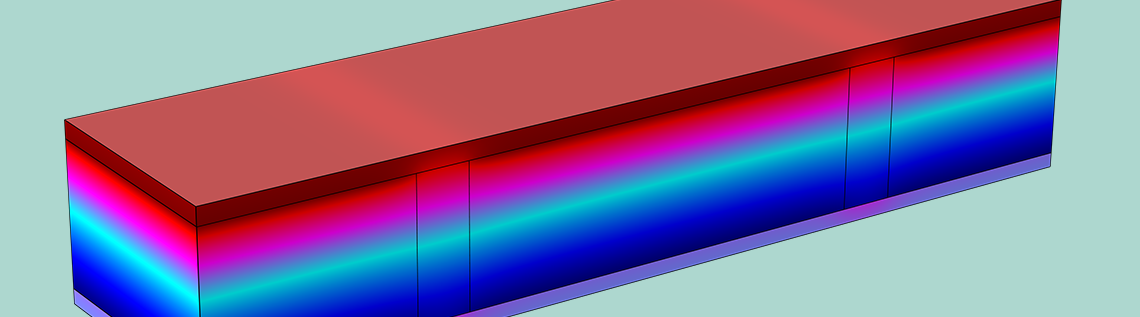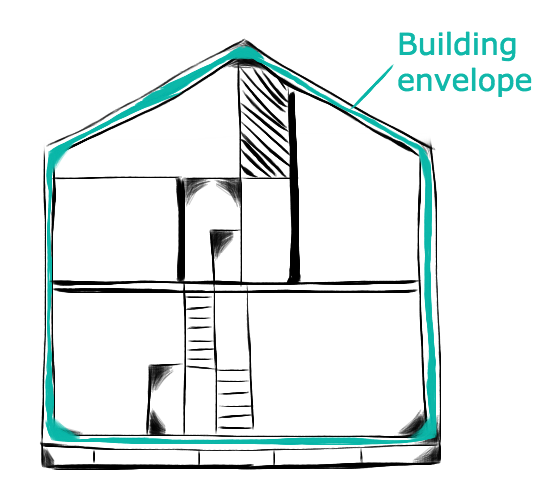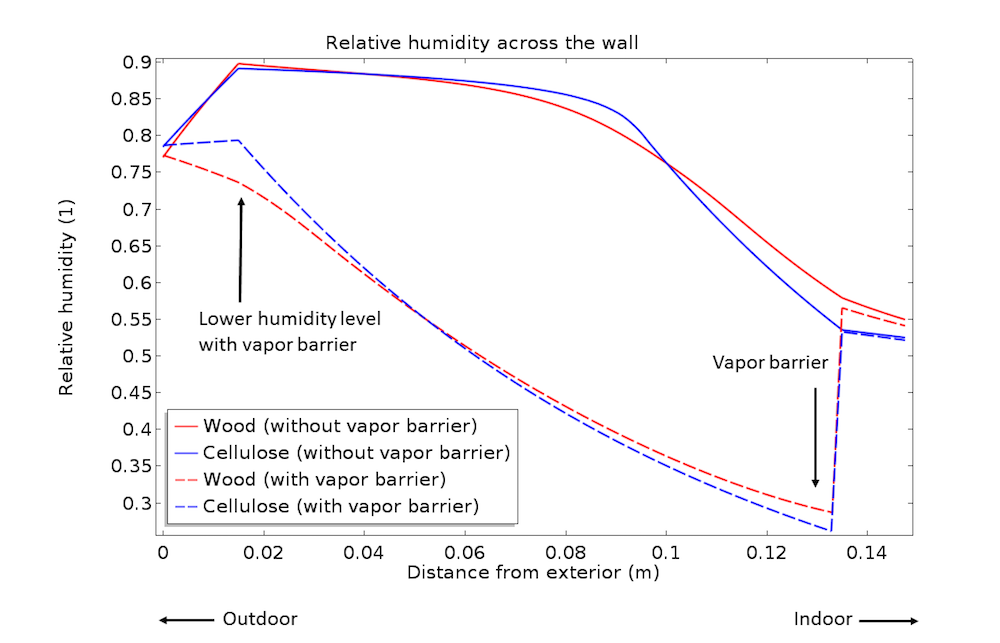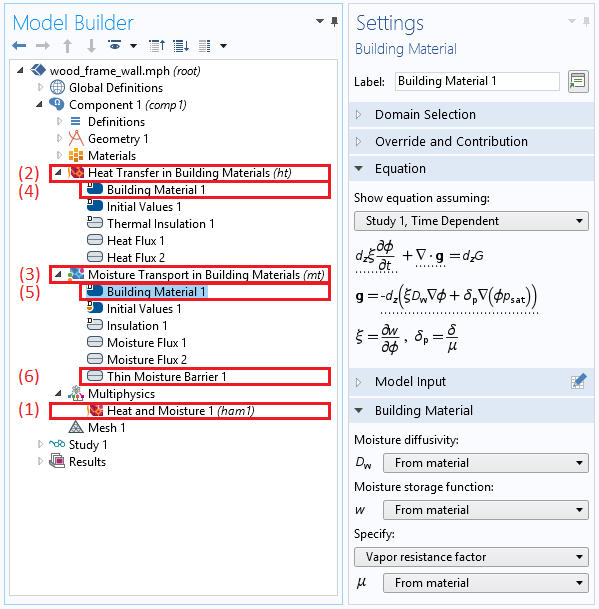# 如何使用 COMSOL® 模拟多孔介质中的热湿传递

2017年 6月 14日

### 建筑材料中的热湿传递模型• 最初施工时产生的水分干燥
• 在较温暖的时期，由于水分从外部向内部迁移而产生的凝结
• 在较冷的时期，由于水汽扩散造成的间歇性凝结导致的水分积累\xi\frac
{\partial \phi} {\partial t}
+ \nabla \cdot \left(- \xi D_\textrm
{w} \nabla\phi -\delta_\textrm{p}\nabla\left(\phi p_\textrm{sat}\right)\right) = G

\mathbf{q}= -L_\textrm{V}\delta_\textrm{p}\nabla\left(\phi p_\textrm{sat}\right)

COMSOL 软件传热模块的热湿传递接口增加了：

1. 热湿 耦合节点
2. 建筑材料传热 接口
3. 建筑材料中的水分输送 接口
4. 用于传热的建筑材料 特征
5. 用于水分传输的建筑材料 特征
6. 薄防潮层 特征，用于模拟防潮层### 非饱和多孔介质中的热湿传递耦合建模

g_\textrm{evap} =M_\textrm{v}K(a_\textrm{w}
c_\textrm{sat}-c_\textrm{v})

### 参考文献

Künzel, H. 1995. Simultaneous Heat and Moisture Transport in Building Components. One and two-dimensional calculation using simple parameters. PhD Thesis. Fraunhofer Institute of Building Physics.

### 动手尝试

• 了解本博客中涉及的教程模型